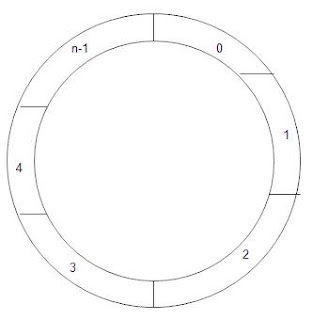Don't miss
Showing posts with label Queue. Show all posts
Showing posts with label Queue. Show all posts

Priority Queue

Priority Queue is same as normal queue like it has a front and rear end,item inserted from rear and deleted from front end but it is more specialised structure as compare to queue.In Priority Queue each items has priority value.It is used in c,c++,java,c# etc.

Type of Priority Queue:-

1)Ascending Priority Queue(Deletion from smallest value).

2)Descending Priority Queue(Deletion from largest value).

Example(Program/implementation/algorithm  for Priority Queue):-

if(rear==50)
cout<<"queue is full";
else
{
q[r]=x;
}
}
void delete()
{
max=q[f];
for(i=f;i<r;i++)
{
if(q[i]>max)
{
max=q[i];
position=i;
}
}
for(j=position;j>f;j--)
q[j]=q[j-1];
f++;
cout<<"deleted element="<<max;
}
void display()
{

for(int i=f;i<f;i++)
cout<<"\n"<<q[i];
}
};

That's all about Priority Queue in data structure

D-Queue(Double Ended Queue)

D-Queue or De queue or double ended queue is a linear data type or abstract data type where insertion and deletion can be possible at either the front and rear end.It is used in c,c++,java,c# etc.

Type of Deques:-

1)I/o Restricted D-Queue(Insertion from one end but deletion from both ends).

2)O/p Restricted D-Queue(Insertion from both ends but deletion from one end).

Example/implementation/algorithm(Program for D-Queue):-

rear-->insert-->r++-->delete-->r--

front-->delete-->f++-->insert-->f--

void insert(int ch1,int x)

{
if(f==0 && r==50)
cout<<"Queue is Full";
else if(f!=0 && ch1==1)
q[--f]=x;
elseif(ch1==0 && r!=50)
q[r++]=x;
else
cout<<"Not Possible";
}
int delete(int ch1)
{
int p;
if(f==r)
{
f=r=0;
return 999;
}
elseif(ch1==1)
p=q[f++];
return p;
}
p=q[r--];
return p;
}
}
void display()
{
for(int i=f;i<r;i++)
cout<<q[i];
}
};

That's all about D Queue in data structure.

Circular Queue

Circular Queue in data structure are those queue which has a circled shape.Like a circle every rear element joins the front end of node.Circular Queue is used in c,c++,java,c# etc.Circular Queue Diagram

Example/implementation/algorithm of circular queue(Program of array):-

void insert()
{
if(f!=0 && r==50)
{
flag=0;
r=0;
q[r++]=x;
}
elseif((f==r && r==50)//(flag==0 && f==r))
cout<<"Queue is Full";
else
q[r++]=x;
}
int delete()
{
if(f==50)
{flag==1;
f==0;
}
elseif(flag==1 && f==r)
{
cout<<"Queue is Empty";
f=r=0;
}
else return q[f++];
}
void disp()
{
if(flag==1)
{
for(i=f;i<r;i++)

cout<<q[i];
else

for(i=f;i<50;i++)
cout<<q[i];
for(i=0;i<r;i++)
cout<<q[i];
}
}

That's all about Circular Queue in computer science.

Queue(Data Structure)

Queue is a abstract data type which work on FIFO(First In First Out) manner.Queue perform insertion(this operation perform from rear end),deletion operation(this operation perform from front end) in computer.Queue is used in c,c++,java,c# etc.

Type of Queue:-

1)Simple Queue(programming example is given below)

2)Circular Queue
3)D-Queue
4)Priority Queue

Let us take an non-programming example(Real World Example):-Queue of people waiting at reception
Functions which are used in Queue:-

1)enqueue()

2)dequeue()
3)first()
4)IsEmpyt()
5)IsFull()
6)Length()

Example/implementation/algorithm of Simple Queue(Program):-

rear-->insert-->rear++
front-->delete-->front++

Program:-

{
if(rear==50)
cout<<"queue is full";
else
{
q[r]=x;
}
}
void delete()
{
if(f==r)
{
cout<<"queue is empty";
f=r=0;
}
else
cout<<"\n deletef element as<<q[f++]";
}
void display()
{
for(int i=f;i<f;i++)
cout<<"\n"<<q[i];
}
};

That's all about Queue and its definition/meaning in data structure.

What is Stack|Example of Stack

Stack is a data structure or we can say that its a linear data structure which is used to store data element and retrieved data element in LIFO Manner.LIFO stands for Last In First Out.In Stack data element are added,removed from the single end i.e. top of the stack but in queue data element are added,removed from both the ends means insertion from rear end and deletion from front end.In stack we used two terms push and pop.Push means adding element to stack and pop means removing element from stack.

Let us take an non-programming example:-

In above figure We tried to explain how the stack works in our daily life.Balls are added to the top of the stack.The last ball put in the stack is the first ball removed by the user.

top=4

insert-->push-->top++
delete-->pop-->top--

Functions which are used in Stack:-

2)Pop:-Removing element from stack.
3)Clear:-Used to clear all the node in the stack.
4)IsEmpty:-Check "Is Stack is Empty".
5)IsFull:-Check "Is Stack is Full".

Programming Example:-Write a Class Stack program which perform this operations:-

1)Push()

2)Pop()
3)Display()

Program:-

#include<iostream.h>

#include<conio.h>
int top=-1;
class stack
{
int st;
public:
stack()
{
}
void push(int x)
{
if(top==49)
cout<<"Stack is full";
else
st[++top]=x;
}
int pop()
{
if(top==-1)
return -999;
else
return st[top--];
}
void display()
{
if(top==-1)
cout<<"Stack is empty";
else
for(int i=0;i<=top;i++)
{
cout<<"\n"<<st[i];
}
}
};

void main()

{
stack object;
int n,ch;
while(1)
{
clrscr();

cin>>ch;

switch(ch)
{
case 1:top==-1;
cout<<"enter a value";
cin>>n;
object.push(x);
break;

case 2:cout<<object.pop();

break;

case 3:object.display();

break;

case 4:Exit(0);

}
}
getch()
}